• Weisfeiler-Lehman图同构测试及其他 神经网络 分子指纹

Weisfeiler-Lehman图同构测试及其他 Weisfeiler-Lehman Test (WL Test) Boris Weisfeiler and Andrey Lehman, 1968 Graph Isomorphism 一个简单的同构图例子： 1-dimensional WL Test 输入：两个可有节点属性的 ...

Weisfeiler-Lehman图同构测试及其他

Weisfeiler-Lehman Test (WL Test)

Boris Weisfeiler and Andrey Lehman, 1968

Graph Isomorphism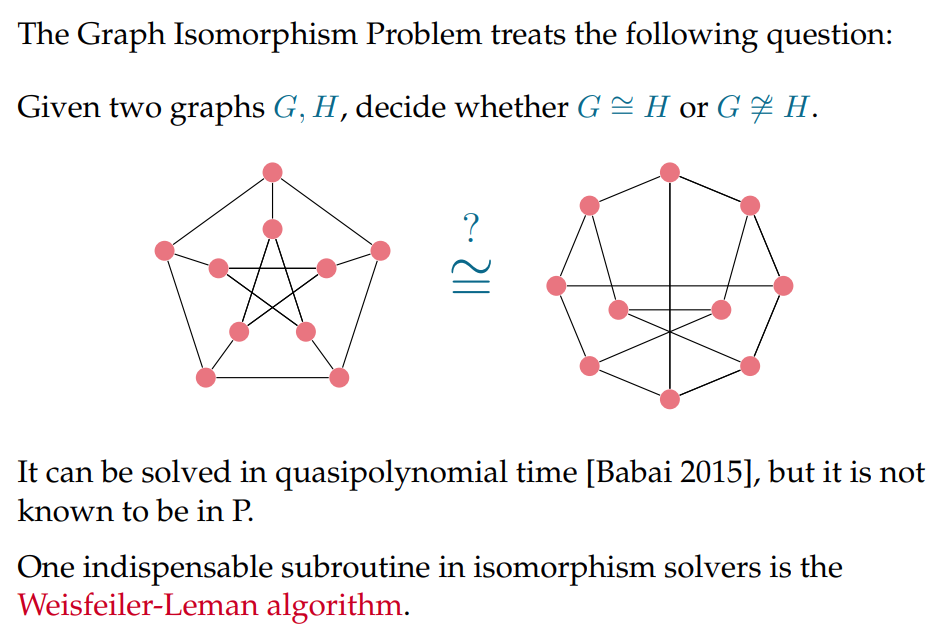一个简单的同构图例子：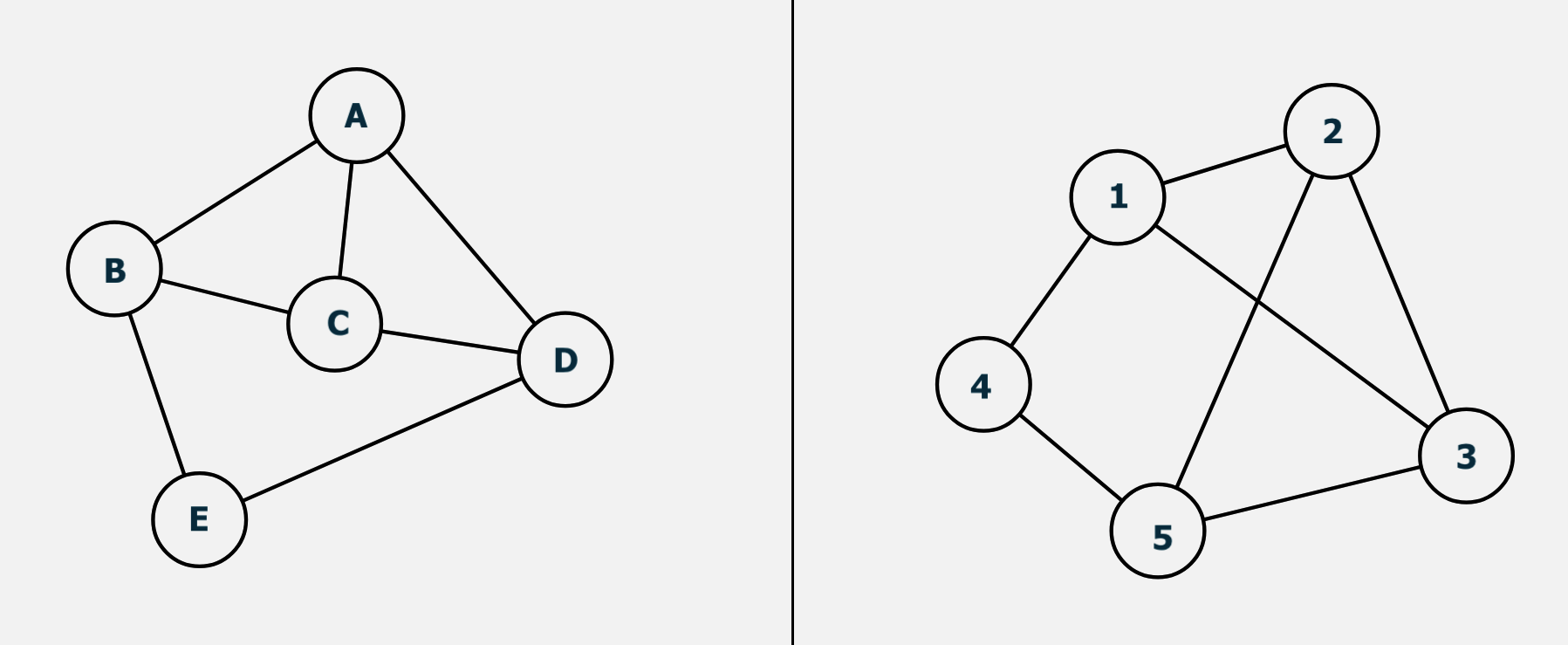1-dimensional WL Test

输入：两个可有节点属性的图

输出：两个图是否同构（满足WL Test是两图同构的必要条件）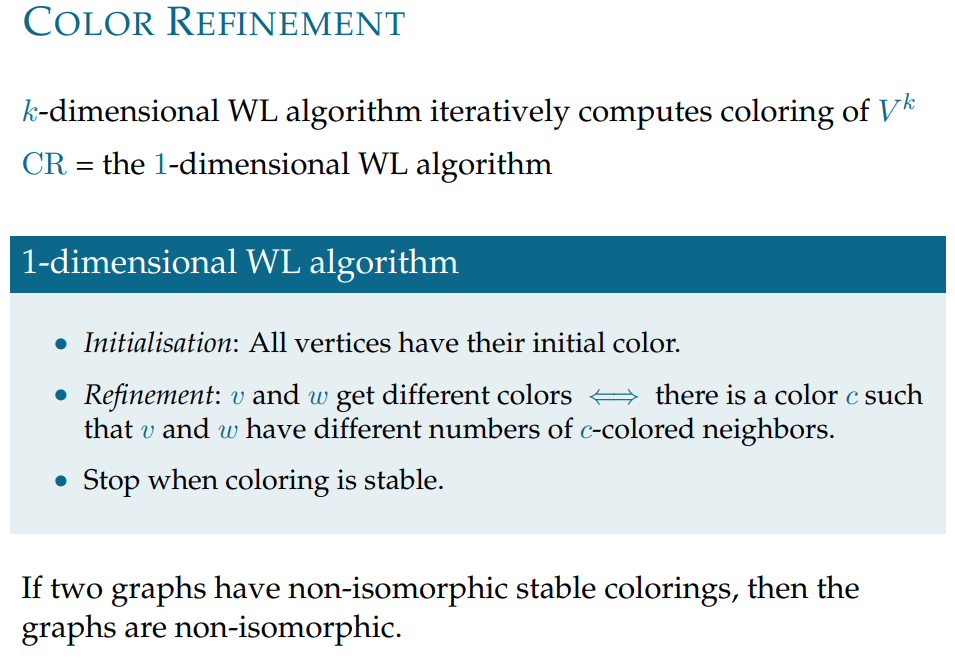• 演示1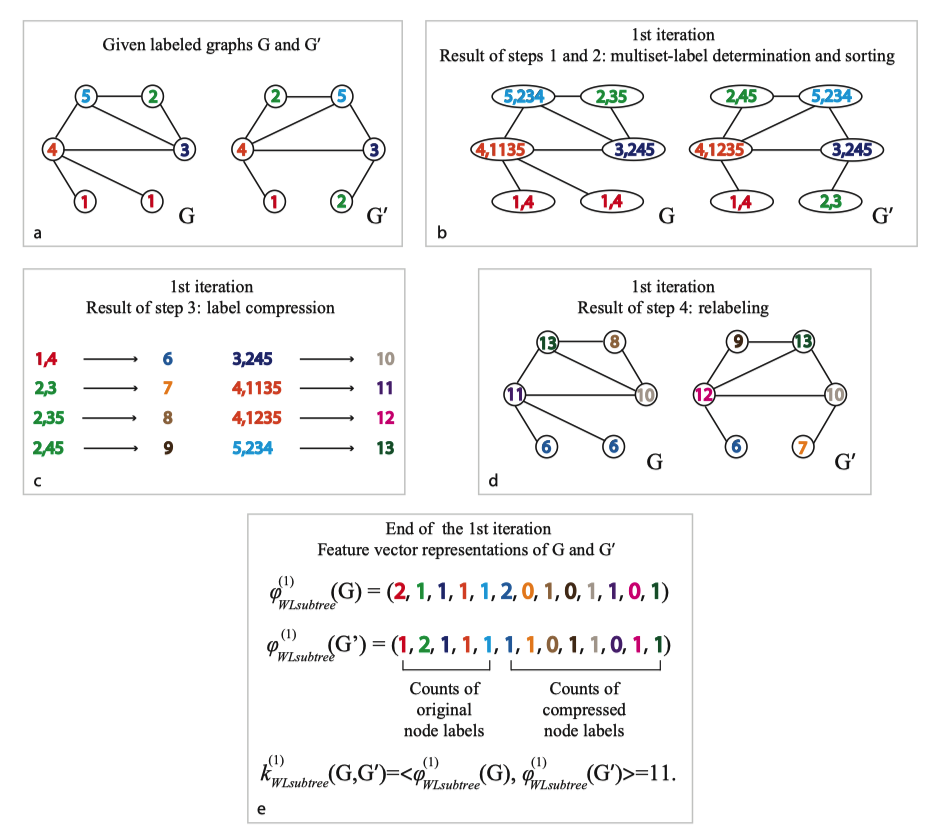• 演示2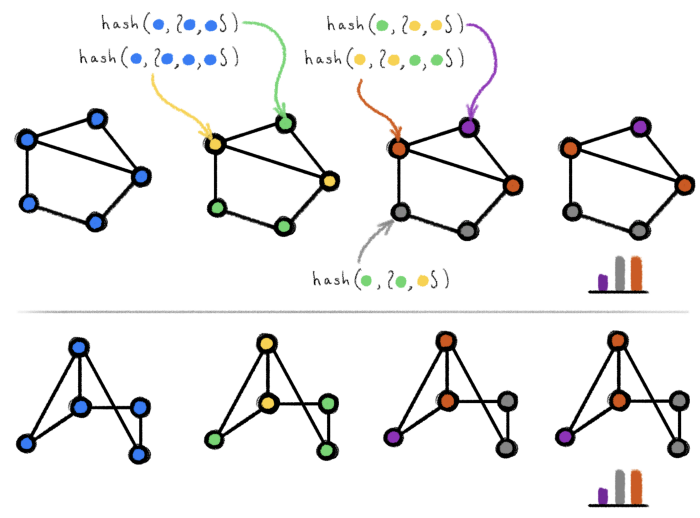• 更加具体的描述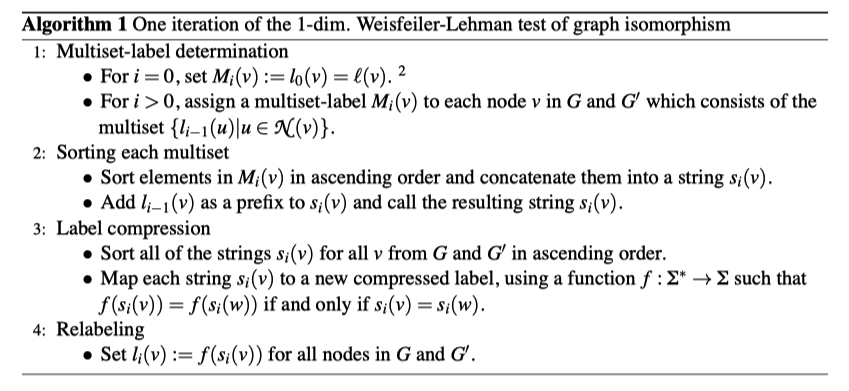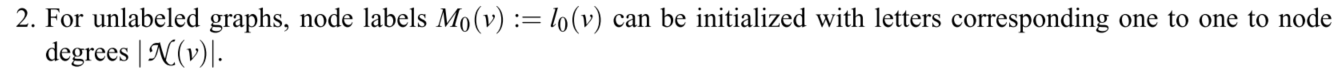• 稳定状态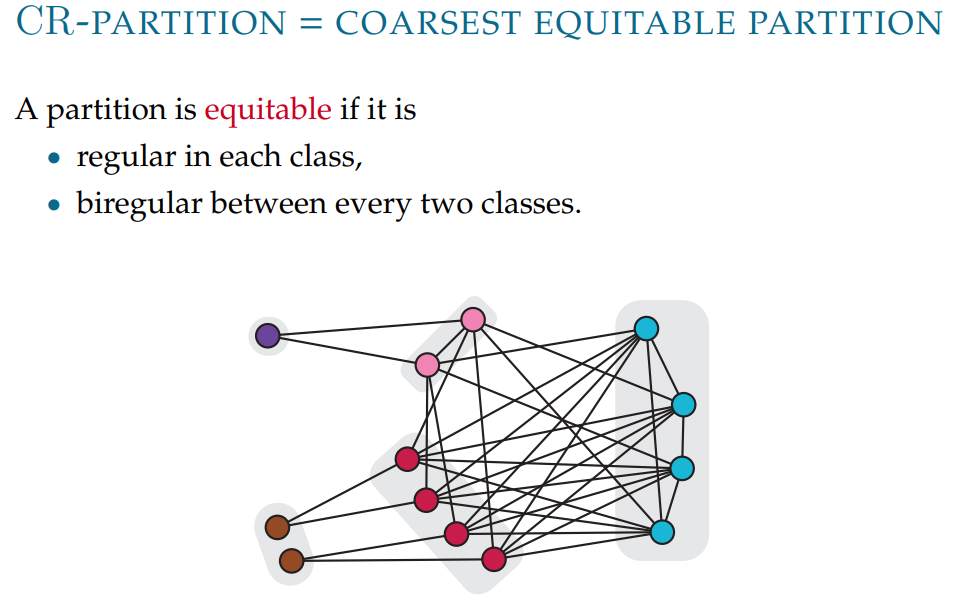• 失效情况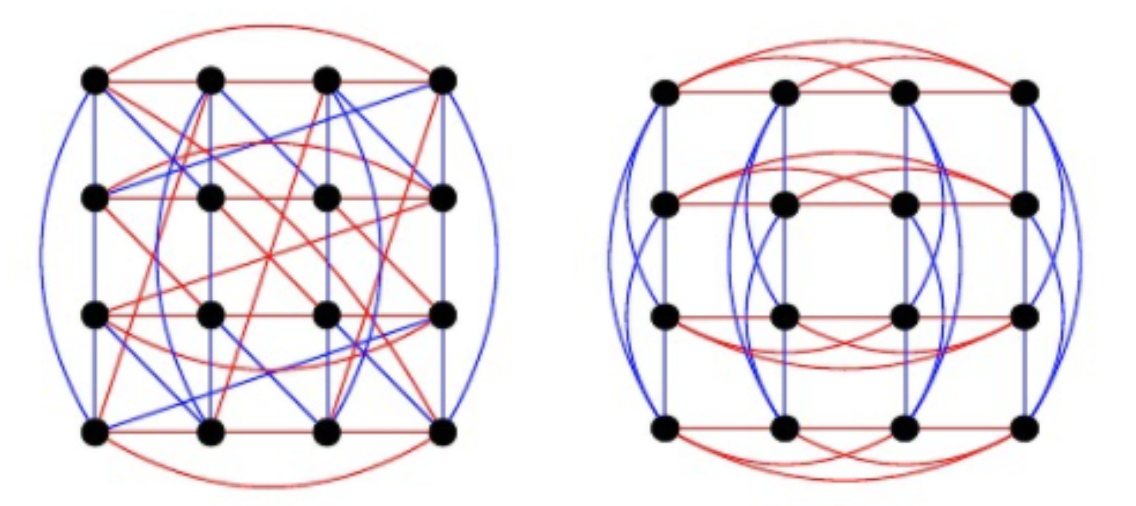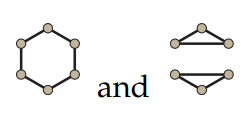注意这里和原ppt不同，2-WL其实应该是无法区分这个六边形和两个三角形的。（一种说法是1-WL和2-WL的能力其实是一样的。）

k-dimensional WL Test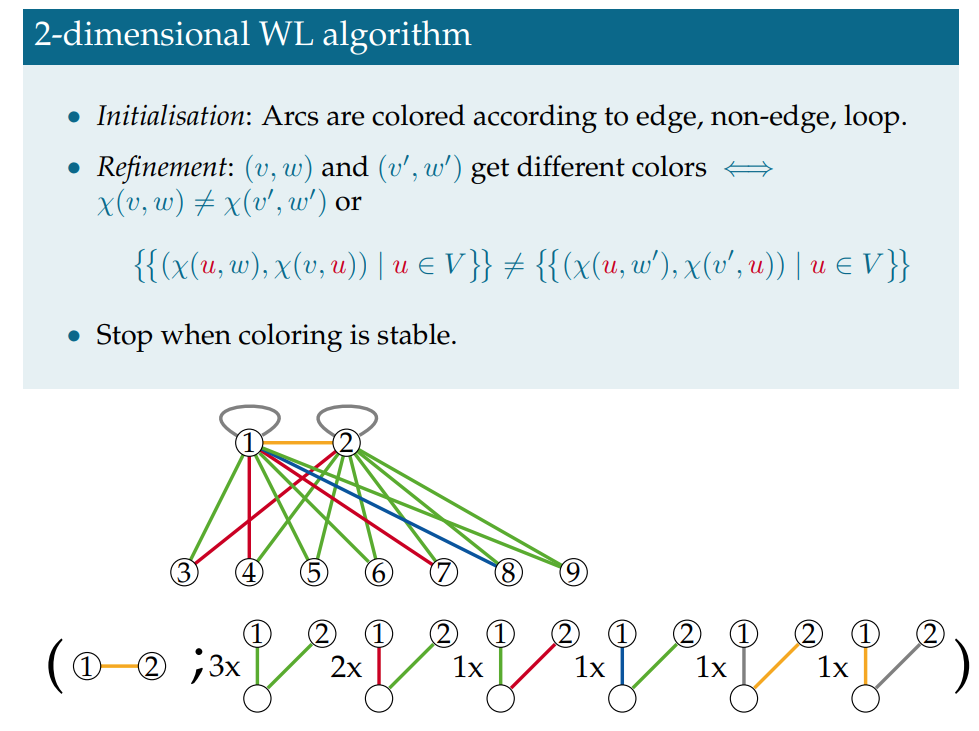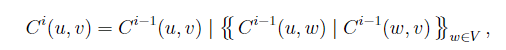3维的WL Test首先枚举图中所有三个点的组合，初始化标签，然后按照类似的方法进行细化。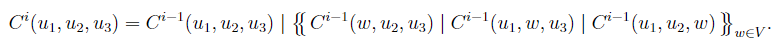k维的WL Test考虑了k个节点的组合。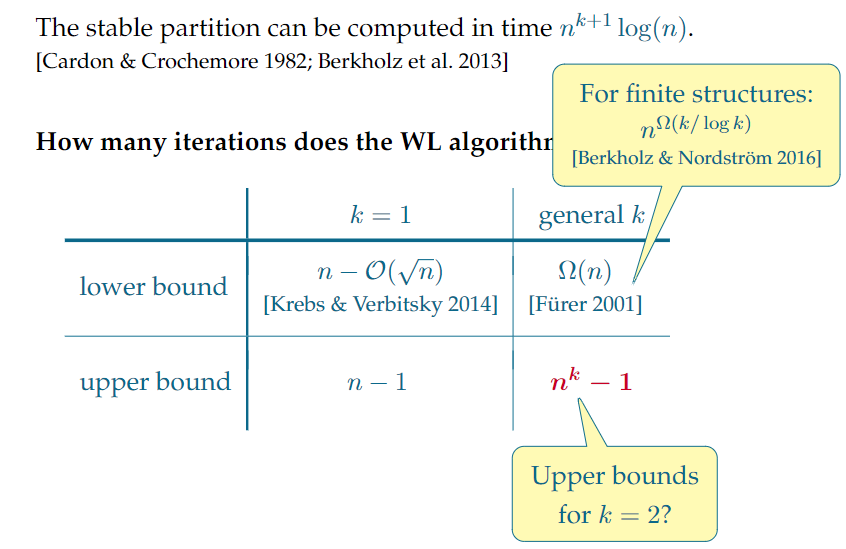Morgan Algorithm

Morgan, 1965

• Chemical Fingerprints (ECFP)

The ECFP generation process has three sequential stages:

1. An initial assignment stage in which each atom has an integer identifier assigned to it.
2. An iterative updating stage in which each atom identifier is updated to reflect the identifiers of each atom’s neighbors, including identification of whether it is a structural duplicate of other features.
3. A duplicate identifier removal stage in which multiple occurrences of the same feature are reduced to a single representative in the final feature list. (The occurrence count may be retained if one requires a set of counts rather than a standard binary fingerprint.)
1. 初始化原子标识符。哈希函数处理非氢原子属性（原子序号、连接性等），得到一个整数
2. 标志符的迭代更新。类似Mogan算法，但是迭代次数是预先设定的，不追求得到1-WL的区分度。
3. 标识符去重。保留所有不同的标识符，压缩到一个比特串中。
• Canonical SMILES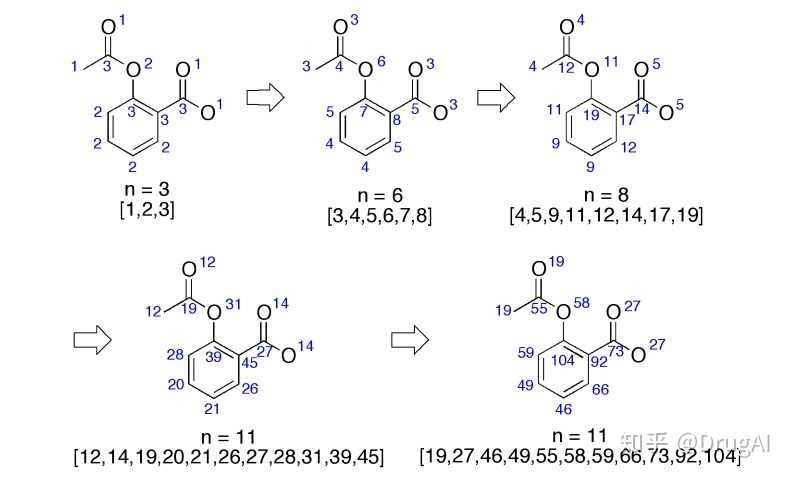利用Mogan算法迭代连通度，直到稳定（更新后连通度直方图形状不变），利用连通度进行排序，得到唯一的SMILES记法。（这种算法是商业化的，所以计算Canonical SMILES要用Daylight软件。）

Message-Passing Neural Network (MPNN)

Weisfeiler-Lehman Netwrok (WLN)

WLN的思想是将1-WL 中离散的呈指数增长的节点标签用嵌入向量代替

用于预测有机分子化学反应，NeurIPS 2017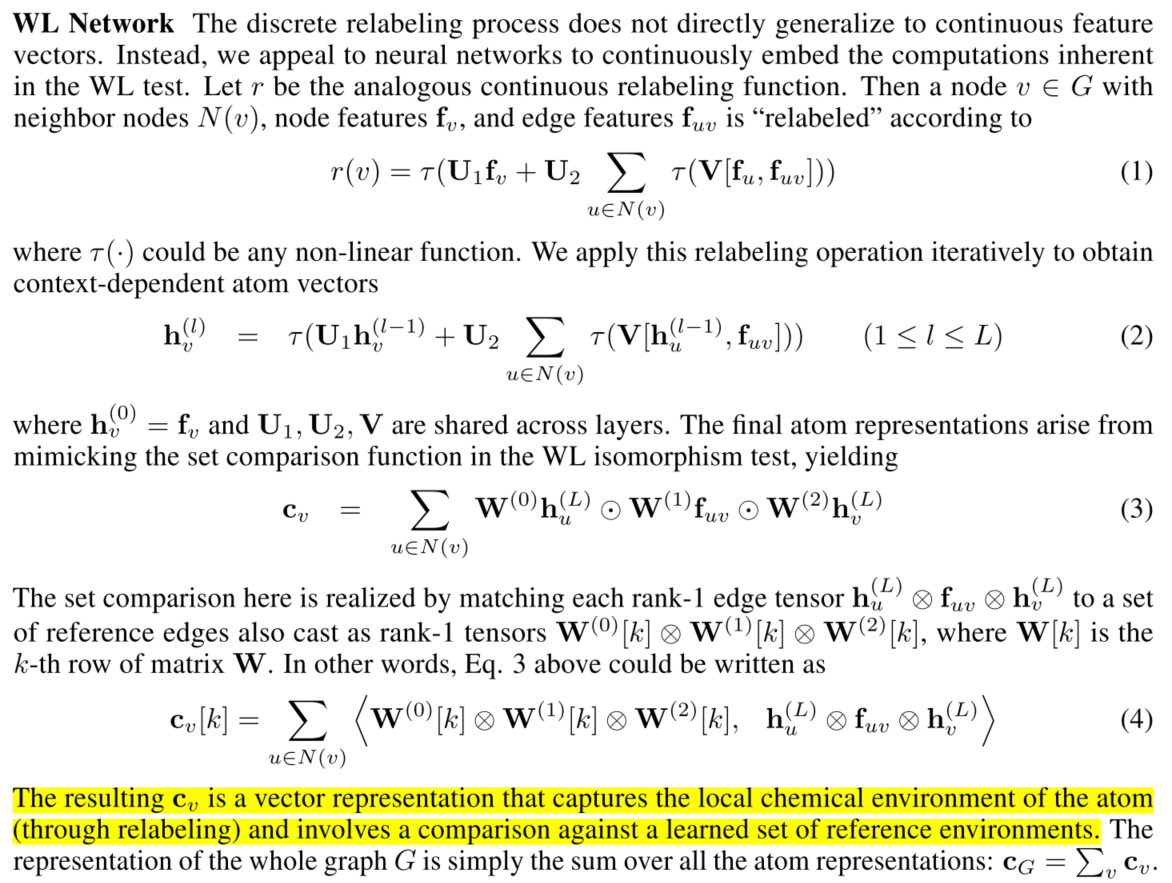MPNN

消息传递网络MPNN是一种聚合邻近点信息的图神经网络框架。

MPNN contains two phases, a message passing phase (namely the propagation step) and a readout phase.

• The message passing phase runs for T times and is defined by message function Mt and vertex update function Ut.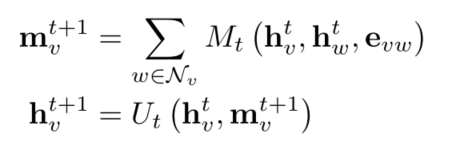where m v t m_v^t is message and e v w e_{vw} is the feature of the edge from node v to w

• The reader phase computes a feature vector for the whole graph using readout function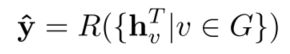How Powerful Are Graph Neural Networks？

ICLR 2019

WL Test & MPNN

• MPNN• 1d WL Test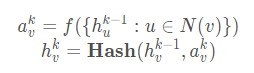1-WL 是MPNN类型的GNN的性能上界

不过利用WL得到的节点特征是离散的，或者说是one hot类型的，不能用于计算图的相似度等。

• 设计合适的更新函数和聚合函数非常重要
• 常用的聚合函数如MAX、MEAN不能处理的一些情况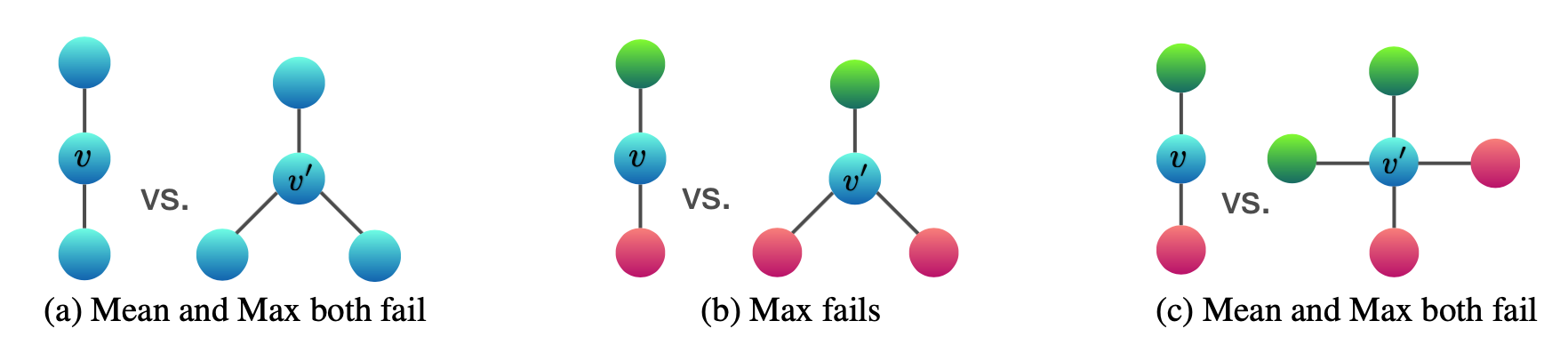聚合函数需要是 单射(injective) 的，即函数不同的输入不能有相同的输出。

Graph Isomorphism Networks (GIN)

• 更新（以及聚合）函数：MLP+SUM
• 存在这样一个函数是单射的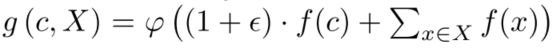• 用MLP拟合 f ϕ f\phi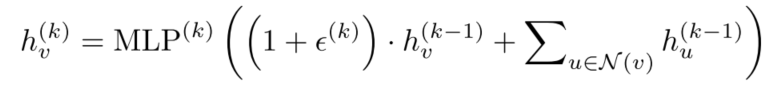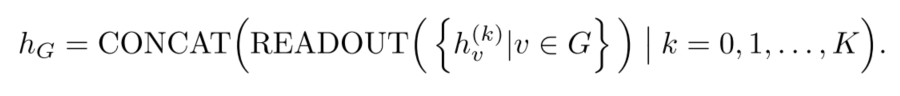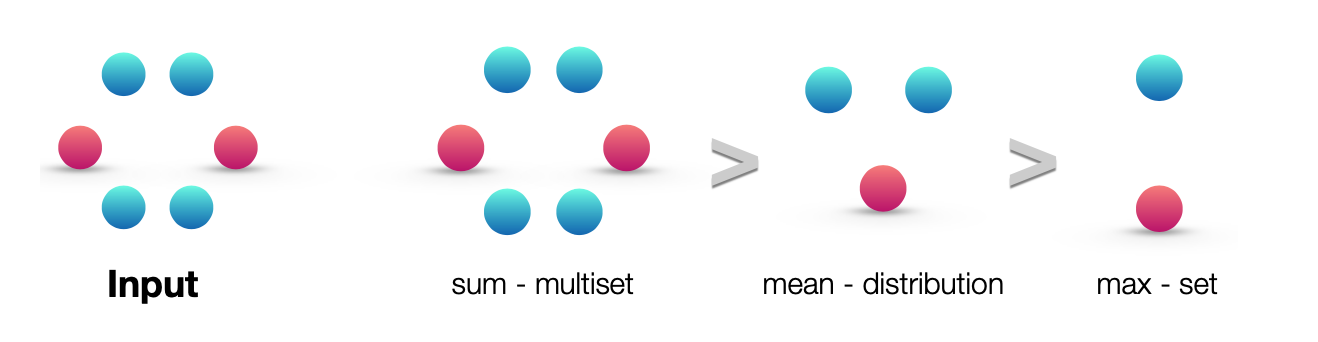实验（图分类 Graph Classification)

训练集：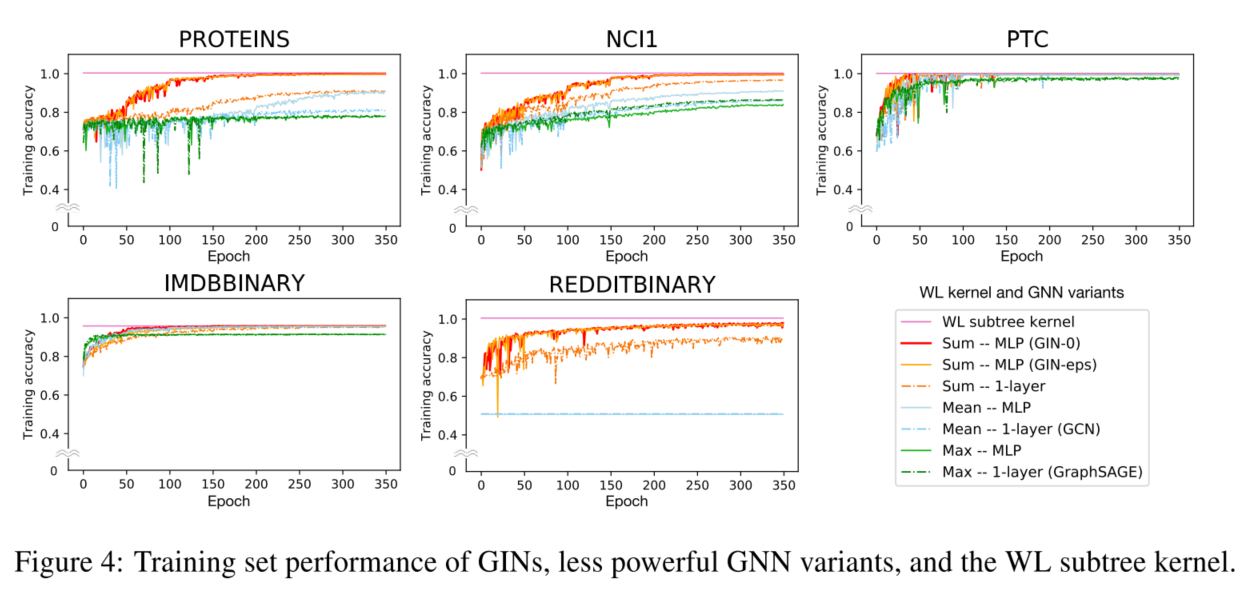测试集：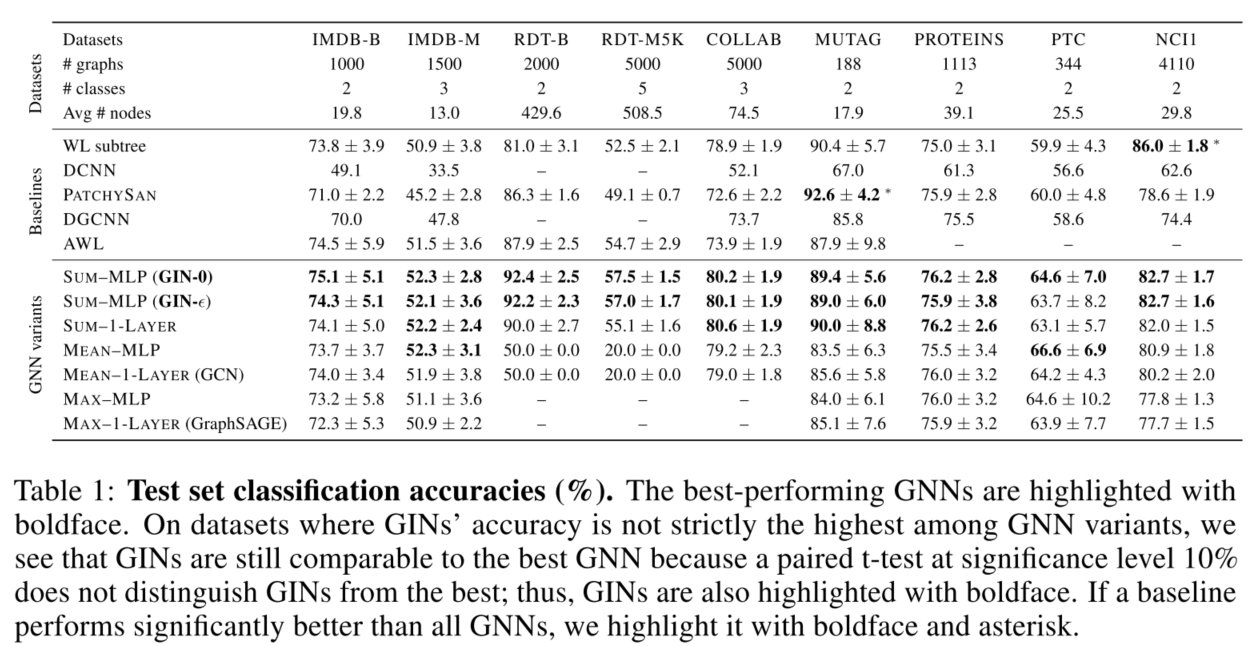Beyond WL

Beyond 1-WL

non local

基于k-WL及各种变种k-WL设计的网络，虽然理论很好但实际效果不佳。

• k-GNNs 需要 O ( n k ) O(n^k) 级别内存
• Invariant Graph Networks (IGN) based on k-order tensors
• 3-WL 级别的IGN有平方级别的复杂度，但较MPNN的线性复杂度还是略显臃肿

Beyond WL

• GSN 在MPNN的基础上，使聚合的信息包括局部图结构（保留了局部性和线性复杂度）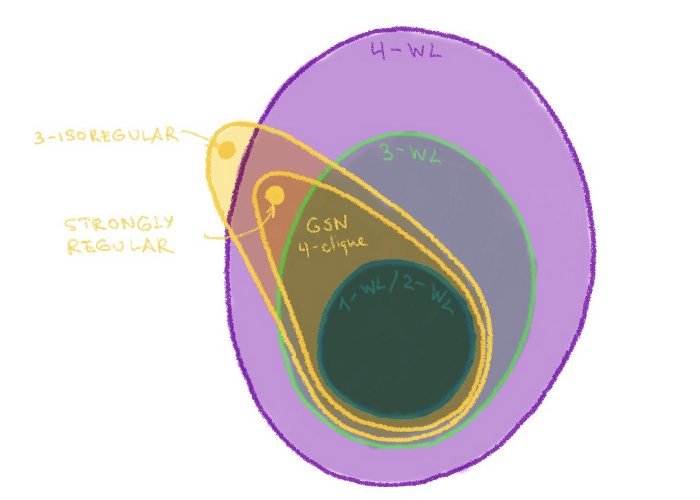• 近似同构，用某种度量来衡量两图的相似性

Reference

Michael Bronstein’s Blog (Recommended)

WL Test

• Combinatorial Properties of the Weisfeiler-Leman Algorithm by Sandra Kiefer
• Weisfeiler-lehman graph kernels
• On Weisfeiler-Leman Invariance: Subgraph Counts and Related Graph Properties

Chemical Fingerprint

WLN

• Predicting organic reaction outcomes with weisfeiler-lehman network NeurIPS2017

MPNN

• Graph neural networks: A review of methods and applications arXiv 2018
• Neural message passing for quantum chemistry arXiv 2017

GIN

• How powerful are graph neural networks? ICLR 2019
展开全文csdn_yi_e 2021-03-22 14:54:55
• 判断图同构大杀器---nauty算法 算法 同构

判断两是否同构是一个经典问题。 nauty算法作为时下较为流行的主流算法，具有效率高，剪枝力度强等优势。当然，在某些特殊情况会失灵。 虽然该算法的概念在上世纪80年代就提出来了，但发展至今，仍然是不可忽略的...

判断两图是否同构是一个经典问题。
nauty算法作为时下较为流行的主流算法，具有效率高，剪枝力度强等优势。当然，在某些特殊情况会失灵。
虽然该算法的概念在上世纪80年代就提出来了，但发展至今，仍然是不可忽略的一种方法。

本人翻遍了中文互联网，没找到详细相关介绍，在stack overflow上边找到了一个问答，顺着帖子的回复找到了算法原作者自建的网站，如获至宝。
再结合离散数学，看懂了这个算法的大致流程。总结如下：

nauty算法：判断两个图是否同构。
思路：
①设置一套编号系统，给两个图进行编号，如果两个图对应的正则编号是一样的，两图同构。

②设置编号系统：
1）对图进行划分，使得任意一个节点的不同颜色的邻接节点的个数相同。
2）对于包含节点个数大于1的子集合再划分，一直化到所有子集合元素个数为1。
3）回溯，得到第二个划分。对比两个划分方式，得到置换群信息，利用这一信息去剪枝。
4）回溯，得到第三个划分，再得到一些置换群的信息，进一步剪枝。
5）最终存留的划分结果即是这个图的可行的命名方式。选择其中最大字典的一种作为正则编号，与另外一个图进行比对。

③剪枝过程中还利用了【节点不变量】信息。具体来说，用一个函数评价某节点的【节点不变量】。
在同一深度下，某节点的函数值相较另一个节点高，则正则编号将出现在该节点的子树下。
在同一深度下，某节点的函数值与另一个节点不相等，则二者的子树不一样。
两节点在某一群作用下相同，则两节点的子树有对应相等值。

部分理解存在偏差，对该算法感兴趣的朋友可以去作者自建的网站了解详情，里边有漂亮的PPT，排版也很好。

另外，nauty算法剪枝的核心是求解自同构群，这一过程在网站的search tree那一栏也有详尽的说明（类似PPT），结合基础群论知识，很容易理解。

展开全文frank_haha 2021-06-22 20:57:12
• 同构 先解释啥叫同构： “ 设两个G1=(V1,E1)和G2(V2,G2)。a,b∈V1,c,d∈V2，若存在一个一对一和满射函数：f，使得f(a)=c，f(b)=d，并且a和b相邻时，c与d也相邻。 设两个G_1=(V_1,E_1)和G_2(V_2,G_2)。\\ a,b...

图的同构

先解释啥叫同构：

设 两 个 图 G 1 = ( V 1 , E 1 ) 和 G 2 ( V 2 , G 2 ) 。 a , b ∈ V 1 , c , d ∈ V 2 ， 若 存 在 一 个 一 对 一 和 满 射 函 数 ： f ， 使 得 f ( a ) = c ， f ( b ) = d ， 并 且 a 和 b 相 邻 时 ， c 与 d 也 相 邻 。 设两个图G_1=(V_1,E_1)和G_2(V_2,G_2)。\\ a,b \in V_1,c,d \in V_2，若存在一个一对一和满射函数：f，\\ 使得f(a)=c，f(b)=d，并且a和b相邻时，c与d也相邻。

如果那个函数存在，则我们叫G1G2同构的，对应的函数叫做同构。

比如下面两张图：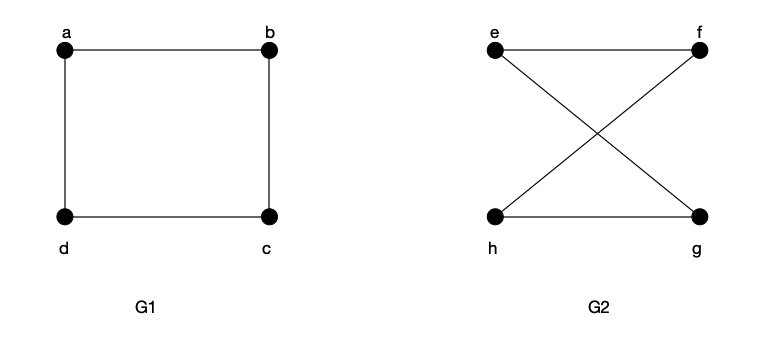对应的f函数如下所示：
f ( a ) = e f ( b ) = f f ( c ) = h f ( d ) = g f(a)=e \\ f(b)=f \\ f(c)=h \\ f(d)=g
因为这样的函数存在，所以可以说G1G2是同构的。或者换成下面的图片会更好理解：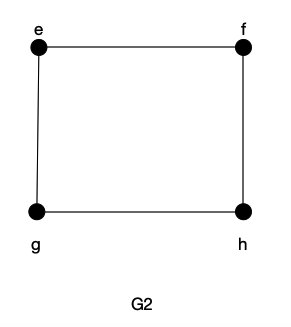这里只是介绍同构的概念，具体判定同构的算法就没有了。

展开全文YQXLLWY 2021-02-09 16:17:48
• 图的同构识别算法——C++代码实现 c++ 数据结构

同构识别： 给定的两个邻接矩阵，判断其三个必要非充分条件： ①结点数目相同 ②变数相同 ③度数相同的结点数相同 以①②③为前提进行矩阵变换，看给定的两个矩阵中，其中的一个矩阵是否能变换为另一个矩阵;...

图的同构识别：

给定的两个邻接矩阵，判断其三个必要非充分条件：
①结点数目相同
②变数相同
③度数相同的结点数相同
以①②③为前提进行矩阵变换，看给定的两个矩阵中，其中的一个矩阵是否能变换为另一个矩阵;

阅读文章前需要知道一个概念:
邻接矩阵中的结点的次序是有实际意义的，当结点进行行变换的时候，必须对其对应的列也进行变换
温馨提示：
博客前两片示例代码段是有小瑕疵的

实现代码和说明:

#include<iostream>
#include<stdlib.h>
#define MAX 100
using namespace std;

int points;             //邻接矩阵的顶点个数（即矩阵阶数）
int edges;              //邻接矩阵的边的条数（即邻接矩阵非零点个数/2）
int Matrix[MAX][MAX];   //矩阵
int weight[MAX];        //行和度数的集合
};

//三个必要条件 ① 结点数相同 ②边数相同 ③ 度数相同的结点数相同

// (行进行交换)
//行位置交换函数，返回true为正常交换,这里的行列交换都是针对于图A的
bool SwapRows(int i,int j){
int k;
//进行 行交换
for(k=0;k<A.points;k++){//矩阵的i 行和j行进行交换
int temp;
temp = A.Matrix[i][k];
A.Matrix[i][k]= A.Matrix[j][k];
A.Matrix[j][k]= temp;
}
int temp;
//行交换了，度也要跟着交换
//这种操作，相当于把点进行移动，移动到某个位置，
//相当于三维世界的直接拖动，即：他的点本来是堆叠起来的(或者次序不当)，然后我们将他的点散开(或者移动)，重新按照某种规则进行摆放(这种规则让当前图的结构(矩阵)趋近于目标图)
//行交换完毕后其度也要记得改变
temp =A.weight[i];
A.weight[i]= A.weight[j];
A.weight[j]= temp;
return true;
}

//(列交换)

//列位置交换函数，返回true为正常交换，false为无法交换
bool  SwapColumns(int currentLayer,int i,int j){//为什么三个参数呢 : 为了保持前面修改的趋势 不被改变
int k;
//判断是否能交换

//这个循环的意思是，我之前从第一行开始，我们的图A尽量与图B相同，然后，currentLayer是当前的层次
//可以理解为同步到当前层次了，然后，如果我们的列  交换，如果它们不相同,则 会破坏我们之前 尽量 与 图B 结构 靠近 的这个趋势的话,我们是不能让它继续进行下去的
//因为如果我们 前面  图A和图B同步了第一行，然后图A在与图B的其他行进行 同步的时候，发现，如果交换的结果会影响到之前的同步结果的话
//那么这样就没法同构了,也就是这两个矩阵，不可能相同
for(k=0;k<currentLayer;k++){//第i列和第j列进行调换

if(A.Matrix[k][i]!=A.Matrix[k][j]){
//无法交换，因为交换后会影响先前调整的结果，故而不同构
return false;
}
}
//进行列交换
for(k=0;k<A.points;k++){
int temp;
temp =A.Matrix[k][i];
A.Matrix[k][i]= A.Matrix[k][j];
A.Matrix[k][j]= temp;
}
return true;
}

//用于快速排序的比较算法
int cmp( const void *a , const void *b ){
return *(int *)a - *(int *)b;
}

int main(){
cout<<"请输入两个图的阶数（顶点数）："<<endl;
cin>>A.points>>B.points;

//判断第一个必要条件

if(A.points!=B.points){
cout<<"阶数不同！不同构！"<<endl;
return 0;
}

cout<<"请输入第1个图的邻接矩阵："<<endl;
A.edges = 0;
B.edges = 0;

//用邻接矩阵方式输入A、B矩阵
int i,j,k,y;
for(i=0;i<A.points;i++){
for(j=0;j<A.points;j++){
cin>>A.Matrix[i][j];
if(A.Matrix[i][j]==1){
A.edges++;
}
}
}

cout<<"请输入第2个图的邻接矩阵："<<endl;
for(i=0;i<B.points;i++){
for(j=0;j<B.points;j++){
cin>>B.Matrix[i][j];
if(B.Matrix[i][j]==1){
B.edges++;
}
}
}

//判断第二个必要条件

if(A.edges!=B.edges){
cout<<"边的条数不同！不同构！"<<endl;
return 0;
}

//因为是邻接矩阵，所以边的条数（即邻接矩阵非零点个数/2）
//在给边进行赋值的时候，我们在二维 矩阵的值是1的时候都给边+1了，因为是无向图，G[i][j]和G[j][i] 是一样的，因此要/2
A.edges =A.edges/2;
B.edges =B.edges/2;
int Aweight[MAX];//MAX==100
int Bweight[MAX];

//判断第三个必要条件
int x=0;
for(k=0;k<A.points;k++){//A图 共有 point 个点，然后对这些点的度数进行计算
int count=0;//初始化度为0
for(y=0;y<A.points;y++){
if(A.Matrix[k][y]==1){ //有边，度+1,这里不用考虑 要/2 ,因为是针对当前点k而言的,A.Matrix[k][y]==1就说明 k对于y点(变点)而言有边
count++;//度+1
}
}
Aweight[x]= count;//遍历完说有点，统计度后,将其记录在一个一维数组中
A.weight[x++]=count;//当然，需要将当前的度记录在A 数据结构中的 weight数组中，然后x+1;

}
qsort(Aweight,A.points,sizeof(Aweight),cmp);
//调用系统快速排序算法
//进行排序的意义是: 因为 第一个点的度是不确定的，因此，我们值能将这个数组进行从小到大（或者从大到小）进行排序，排序完后，数组就是有规律的了
//然后将 B图 记录 点度数的数组也进行从小到大（或者从大到小）进行排序，排序完后，看是否满足 :
//同构图的三个必要条件中的第三个条件:度数相同的节点个数相同

x=0;
//对矩阵B也进行相同的操作
for(k=0;k<B.points;k++){
int count=0;
for(y=0;y<B.points;y++){
if(B.Matrix[k][y]==1){
count++;
}
}
Bweight[x]= count;
B.weight[x++]=count;
}
qsort(Bweight,B.points,sizeof(Bweight),cmp);//调用系统快速排序算法
//判断是否满足第三个条件
for(k=0;k<A.points;k++){
if(Aweight[k]!=Bweight[k]){
cout<<"边的度数不同！不同构！"<<endl;
return 0;
}
}

//进行矩阵变换

//三个条件都满足，则进行最后的验证操作:将第一个图的矩阵进行变换，让其结构趋近于第二个图
//并且如果操作过程没有被因为   行列交换操作 判断出错而打断(就是不能行列交换，如何行列交换都无法变换成第二个图,进而被打断)

//调整A矩阵成B  请注意:以下操作 列交换 必定伴随着 行的交换 为什么呢: 因为，虽然矩阵的行和列 之间没有太大的关联，即便行交换和列交换并不会改变其点之间的映射关系
//也没有说 行交换后列必须得交换，但是，在表示图的矩阵中，点的次序是有含义的;
for(i=0;i<B.points;i++){
for(j=i;j<A.points;j++){
//找到度相同
//对度数相同的结点进行行交换

if(B.weight[i] == A.weight[j]){//注意，这里是B的度等于 A的度的时候
//进行行交换
if(i!=j){//如果i!=j就交换，否则跳过(不用交换，换了和没换一样)
SwapRows(i,j);//先交换行  注意:行进行交换了之后，对应的列也必须跟着变化(可以理解为行和列结点的顺序必须保持一致)
}

//进行列交换 从上往下,以 i 为行 不断的 向第二个图的结构靠近
if(i!=j){

if(SwapColumns(i,i,j)==false){//交换列
cout<<"无法调整成相同的邻接矩阵！不同构！"<<endl;
return 0;
}

int list[MAX];
x=0;
//判断非零顶点所处列的位置是否相同

//两个for循环是在i!=j的情况下执行的

for(k=0;k<A.points;k++){//找出位置不同的点放入list
if(A.Matrix[i][k]!=B.Matrix[i][k]){//找出A图中与B不相同的位置，记录在list中
list[x]=k;//记录不同的列
x=x+1;
}
}

for(k=0;k<x;k=k+2){
if(SwapColumns(i,list[k],list[k+1])==false){//列交换 伴随着 行交换
//0 1/2 3/4 5
cout<<"无法调整成相同的邻接矩阵！不同构！"<<endl;
return 0;
}//循环交换列
SwapRows(list[k],list[k+1]);//循环交换行
}
}

break;
}
}
}
cout<<"经过检测，两图同构！"<<endl;
return 0;
}

举例:
图G和图G’其矩阵的变换过程如下图: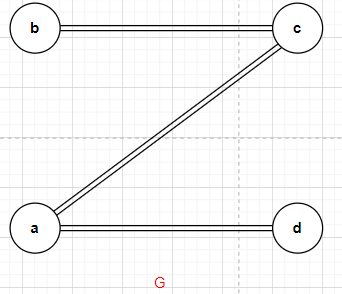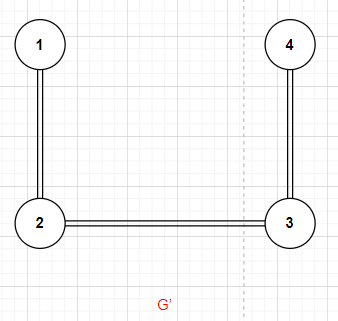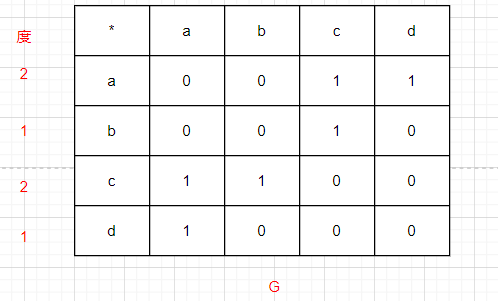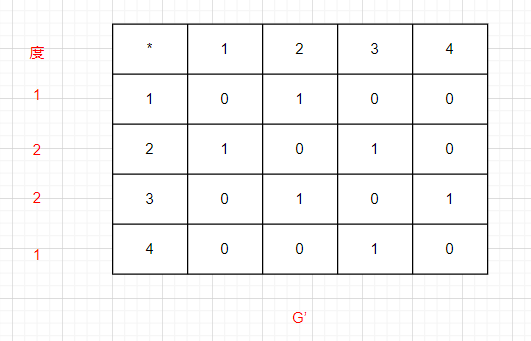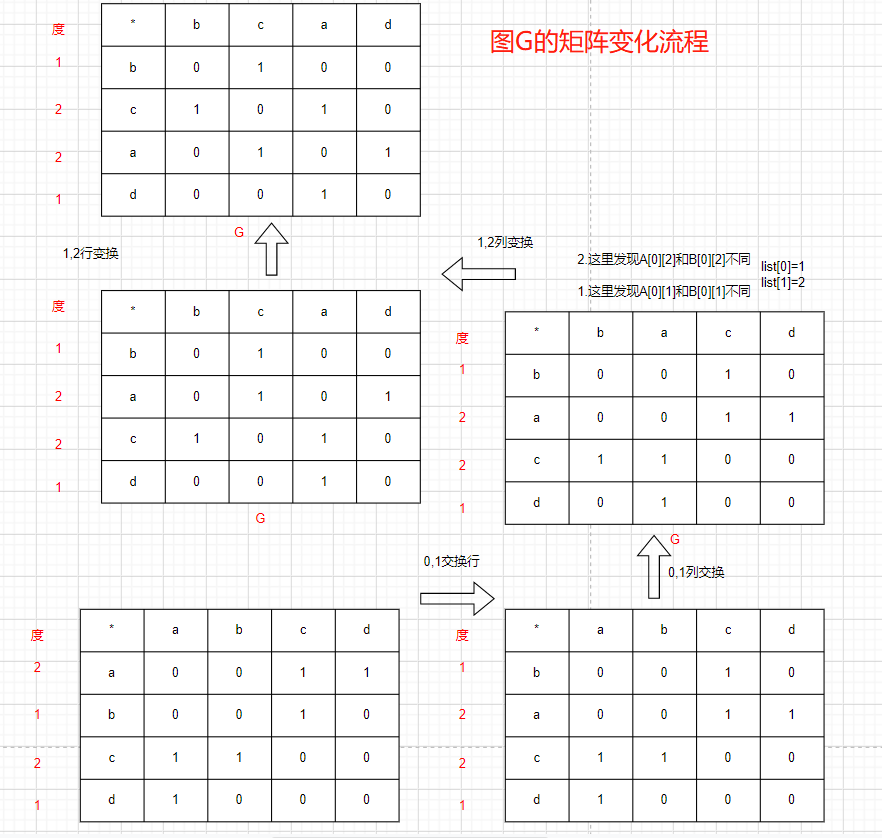最终图G通过移动点的位置，有了与目标图一样的结构，并且其可视化为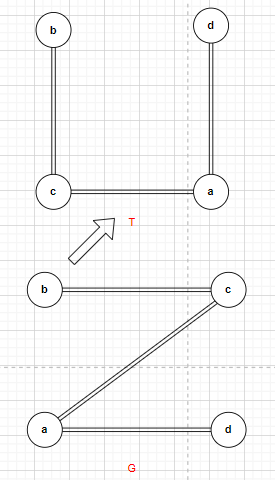然后对比目标图G‘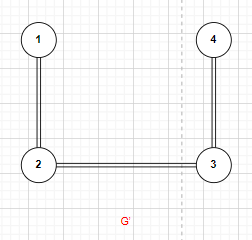我们可以发现，只要变换图G将其结点b与1对应，c与2对应，a与3对应，d与4对应的时候，其结构就可以清楚的看出来两个图的一样的，也就是同构的，其邻接矩阵也是相同的，邻接矩阵的行列也是这样的映射关系
b:1
c:2
a:3
d:4
因此，应了前文那句话，邻接矩阵中的结点的次序是有实际意义的，当结点进行行变换的时候，必须对其对应的列也进行变换.

代码存在的缺点:

对于同构图G’和G‘’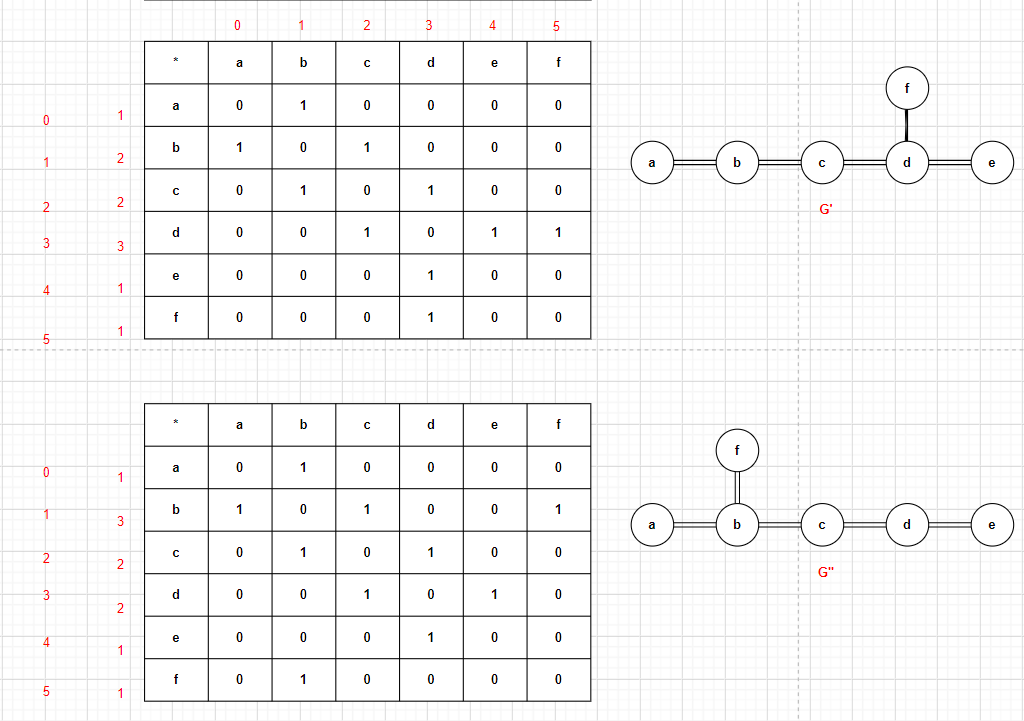其运行结果：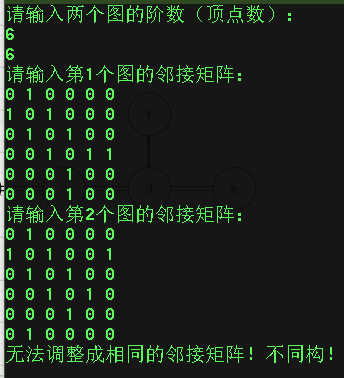对代码进行优化、改进

(其实还是有问题😓😓😓，读者可以忽略改进的代码，直接跳到最后看没有正确的代码,因为代码记录了我对图的同构的理解的过程，因此，不删掉这两段错误的代码)
这是以上代码存在的问题，现对以上代码做优化、改进；
思路如下:
①我们对图G的结构进行调整:
让其每一行的度 调整至G‘,也就是对图G的点，也就是在矩阵中对行列进行移动;
②调整完毕,立刻检查两个矩阵是否相同,若不同，从上往下，调换度相同的结点，遍历所有的可能，每次调换完毕，都检查一次，看是否两个矩阵相同
因此，对函数添加如下代码:
①:
一个中间数组C,(如果这种初始判定条件下，仍然返回false的话，进行后续的判定，而后续的判定需要一个最初始状态的数组A)
注意，这个数组必须也初始化与A相同的度
(不初始化就为0无法判断是否同构)

②:
让第一个图的矩阵的度和第二个图的度保持一致的函数to_be_similar():

void to_be_similar(){
for(i=0;i<B.points;i++){
for(j=i;j<C.points;j++){
if(B.weight[i] == C.weight[j]){//注意，这里是B的度等于 A的度的时候
//进行行交换
if(i!=j){//如果i!=j就交换，否则跳过(不用交换，换了和没换一样)
SwapRows(i,j);//先交换行  注意:行进行交换了之后，对应的列也必须跟着变化(可以理解为行和列结点的顺序必须保持一致)
SwapColumns(0,i,j);//行变化一定伴随列变化
}
}
}
}
}//执行完毕这个函数，那么，这两个矩阵的度的结构就一样了
//(C矩阵度的赋值并不在这里哦)

③:判定函数Judge():

bool Judge(){
for(i =0; i <C.points;i++){
for(j=0; j <B.points;j++){
if(C.Matrix[i][j]!=B.Matrix[i][j])
return false;
}
}
return true;
}

④交换行中度相同的函数并且行交换后列也交换，在交换前判定，交换完毕判定的函数SwapColumnsAndRowsAndJudge():

bool SwapColumnsAndRowsAndJudge(){//直接根据点的度相同，进行列交换 每次交换完行列，都要进行判定：两个矩阵是否相同
for(int x=0;x<C.points;x++){
//交换前进行判断
if(Judge()){
return true;
}
for(y=x;y<C.points;y++){//不用回到之前判断的状态了，所以这里y=x
if(x!=y&&C.weight[x]==C.weight[y]){//&&x!=y
SwapRowsTwo(x,y);
SwapColumnsTwo(x,y);
}
if(Judge()){
return true;
}
}
return false;
}

}

⑤新增加的两个行列置换函数(直接换)
SwapRowsTwo():

bool SwapRowsTwo(int i,int j){//改进代码
int k;

for(k=0;k<C.points;k++){//矩阵的i 行和j行进行交换
int temp;
temp = C.Matrix[i][k];
C.Matrix[i][k]= C.Matrix[j][k];
C.Matrix[j][k]= temp;
}
int temp;
temp =C.weight[i];
C.weight[i]= C.weight[j];
C.weight[j]= temp;
return true;
}

⑥SwapColumnsTwo():

SwapColumnsTwo(int i,int j){//改进代码
int k;
for(k=0;k<C.points;k++){
int temp;
temp =C.Matrix[k][i];
C.Matrix[k][i]= C.Matrix[k][j];
C.Matrix[k][j]= temp;
}
return true;
}

完整代码如下:

#include<iostream>
#include<stdlib.h>
#define MAX 100
using namespace std;

int points;             //邻接矩阵的顶点个数（即矩阵阶数）
int edges;              //邻接矩阵的边的条数（即邻接矩阵非零点个数/2）
int Matrix[MAX][MAX];   //矩阵
int weight[MAX];        //行和度数的集合
};

int i,j,k,y;
//三个必要条件 ① 结点数相同 ②边数相同 ③ 度数相同的结点数相同

// (行进行交换)
//行位置交换函数，返回true为正常交换,这里的行列交换都是针对于图A的
bool SwapRows(int i,int j){
int k;
//进行 行交换
for(k=0;k<A.points;k++){//矩阵的i 行和j行进行交换
int temp;
temp = A.Matrix[i][k];
A.Matrix[i][k]= A.Matrix[j][k];
A.Matrix[j][k]= temp;
}
int temp;
//行交换了，度也要跟着交换
//这种操作，相当于把点进行移动，移动到某个位置，
//相当于三维世界的直接拖动，即：他的点本来是堆叠起来的(或者次序不当)，然后我们将他的点散开(或者移动)，重新按照某种规则进行摆放(这种规则让当前图的结构(矩阵)趋近于目标图)
//行交换完毕后其度也要记得改变
temp =A.weight[i];
A.weight[i]= A.weight[j];
A.weight[j]= temp;
return true;
}

bool SwapRowsTwo(int i,int j){//改进代码
int k;

for(k=0;k<C.points;k++){//矩阵的i 行和j行进行交换
int temp;
temp = C.Matrix[i][k];
C.Matrix[i][k]= C.Matrix[j][k];
C.Matrix[j][k]= temp;
}
int temp;
temp =C.weight[i];
C.weight[i]= C.weight[j];
C.weight[j]= temp;
return true;
}

bool  SwapColumnsTwo(int i,int j){//改进代码
int k;
for(k=0;k<C.points;k++){
int temp;
temp =C.Matrix[k][i];
C.Matrix[k][i]= C.Matrix[k][j];
C.Matrix[k][j]= temp;
}
return true;
}

//(列交换)

//列位置交换函数，返回true为正常交换，false为无法交换
bool  SwapColumns(int currentLayer,int i,int j){//为什么三个参数呢 : 为了保持前面修改的趋势 不被改变
int k;
//判断是否能交换

//这个循环的意思是，我之前从第一行开始，我们的图A尽量与图B相同，然后，currentLayer是当前的层次
//可以理解为同步到当前层次了，然后，如果我们的列  交换，如果它们不相同,则 会破坏我们之前 尽量 与 图B 结构 靠近 的这个趋势的话,我们是不能让它继续进行下去的
//因为如果我们 前面  图A和图B同步了第一行，然后图A在与图B的其他行进行 同步的时候，发现，如果交换的结果会影响到之前的同步结果的话
//那么这样就没法同构了,也就是这两个矩阵，不可能相同
for(k=0;k<currentLayer;k++){//第i列和第j列进行调换

if(A.Matrix[k][i]!=A.Matrix[k][j]){
//无法交换，因为交换后会影响先前调整的结果，故而不同构
return false;
}
}
//进行列交换
for(k=0;k<A.points;k++){
int temp;
temp =A.Matrix[k][i];
A.Matrix[k][i]= A.Matrix[k][j];
A.Matrix[k][j]= temp;
}
return true;
}

void to_be_similar(){
for(i=0;i<B.points;i++){
for(j=i;j<C.points;j++){
if(B.weight[i] == C.weight[j]){//注意，这里是B的度等于 A的度的时候
//进行行交换
if(i!=j){//如果i!=j就交换，否则跳过(不用交换，换了和没换一样)
SwapRowsTwo(i,j);//先交换行  注意:行进行交换了之后，对应的列也必须跟着变化(可以理解为行和列结点的顺序必须保持一致)
SwapColumnsTwo(i,j);//行变化一定伴随列变化

}
break;
}
}
}
}//执行完毕这个函数，那么，这两个矩阵的度的结构就一样了
bool Judge(){
for(i =0; i <C.points;i++){
for(j=0; j <B.points;j++){
if(C.Matrix[i][j]!=B.Matrix[i][j])
return false;
}
}
return true;
}

bool SwapColumnsAndRowsAndJudge(){//直接根据点的度相同，进行列交换 每次交换完行列，都要进行判定：两个矩阵是否相同
for(int x=0;x<C.points;x++){
//交换前进行判断
if(Judge()){
return true;
}
for(y=x;y<C.points;y++){//不用回到之前判断的状态了，所以这里y=x
if(x!=y&&C.weight[x]==C.weight[y]){//&&x!=y
SwapRowsTwo(x,y);
SwapColumnsTwo(x,y);
}
if(Judge()){
return true;
}
}
return false;
}

}

//用于快速排序的比较算法
int cmp( const void *a , const void *b ){
return *(int *)a - *(int *)b;
}

int main(){
cout<<"请输入两个图的阶数（顶点数）："<<endl;
cin>>A.points>>B.points;
C.points=A.points;//注意这里要初始化
//判断第一个必要条件

if(A.points!=B.points){
cout<<"阶数不同！不同构！"<<endl;
return 0;
}

cout<<"请输入第1个图的邻接矩阵："<<endl;
A.edges = 0;
B.edges = 0;

//用邻接矩阵方式输入A、B矩阵

for(i=0;i<A.points;i++){
for(j=0;j<A.points;j++){
cin>>A.Matrix[i][j];
C.Matrix[i][j]=A.Matrix[i][j];//拷贝A到C，用C进行分析
if(A.Matrix[i][j]==1){
A.edges++;
}
}
}

cout<<"请输入第2个图的邻接矩阵："<<endl;
for(i=0;i<B.points;i++){
for(j=0;j<B.points;j++){
cin>>B.Matrix[i][j];
if(B.Matrix[i][j]==1){
B.edges++;
}
}
}

//判断第二个必要条件

if(A.edges!=B.edges){
cout<<"边的条数不同！不同构！"<<endl;
return 0;
}

//因为是邻接矩阵，所以边的条数（即邻接矩阵非零点个数/2）
//在给边进行赋值的时候，我们在二维 矩阵的值是1的时候都给边+1了，因为是无向图，G[i][j]和G[j][i] 是一样的，因此要/2
A.edges =A.edges/2;
B.edges =B.edges/2;
int Aweight[MAX];//MAX==100
int Bweight[MAX];

//判断第三个必要条件
int x=0;
for(k=0;k<A.points;k++){//A图 共有 point 个点，然后对这些点的度数进行计算
int count=0;//初始化度为0
for(y=0;y<A.points;y++){
if(A.Matrix[k][y]==1){ //有边，度+1,这里不用考虑 要/2 ,因为是针对当前点k而言的,A.Matrix[k][y]==1就说明 k对于y点(变点)而言有边
count++;//度+1
}
}
Aweight[x]= count;//遍历完说有点，统计度后,将其记录在一个一维数组中
C.weight[x]= A.weight[x]=count;//当然，需要将当前的度记录在A 数据结构中的 weight数组中，然后x+1;
x=x+1;
}
qsort(Aweight,A.points,sizeof(Aweight),cmp);
//调用系统快速排序算法
//进行排序的意义是: 因为 第一个点的度是不确定的，因此，我们值能将这个数组进行从小到大（或者从大到小）进行排序，排序完后，数组就是有规律的了
//然后将 B图 记录 点度数的数组也进行从小到大（或者从大到小）进行排序，排序完后，看是否满足 :
//同构图的三个必要条件中的第三个条件:度数相同的节点个数相同

x=0;
//对矩阵B也进行相同的操作
for(k=0;k<B.points;k++){
int count=0;
for(y=0;y<B.points;y++){
if(B.Matrix[k][y]==1){
count++;
}
}
Bweight[x]= count;
B.weight[x++]=count;
}
qsort(Bweight,B.points,sizeof(Bweight),cmp);//调用系统快速排序算法
//判断是否满足第三个条件
for(k=0;k<A.points;k++){
if(Aweight[k]!=Bweight[k]){
cout<<"边的度数不同！不同构！"<<endl;
return 0;
}
}
//矩阵可能一开始就是同构的，然后，因为点的次序不同，而导致矩阵不相同，此时，我们只需要将矩阵进行行列交换，遍历所有的可能，看是否能够达到两个矩阵相同的效果

to_be_similar();
if(	SwapColumnsAndRowsAndJudge()){
cout<<"经检测，两个图同构!"<<endl;
return 0;
}

//进行矩阵变换

//三个条件都满足，则进行最后的验证操作:将第一个图的矩阵进行变换，让其结构趋近于第二个图
//并且如果操作过程没有被因为   行列交换操作 判断出错而打断(就是不能行列交换，如何行列交换都无法变换成第二个图,进而被打断)

//调整A矩阵成B  请注意:以下操作 列交换 必定伴随着 行的交换 为什么呢: 因为，虽然矩阵的行和列 之间没有太大的关联，即便行交换和列交换并不会改变其点之间的映射关系
//也没有说 行交换后列必须得交换，但是，在表示图的矩阵中，点的次序是有含义的;
for(i=0;i<B.points;i++){
for(j=i;j<A.points;j++){
//找到度相同
//对度数相同的结点进行行交换

if(B.weight[i] == A.weight[j]){//注意，这里是B的度等于 A的度的时候
//进行行交换
if(i!=j){//如果i!=j就交换，否则跳过(不用交换，换了和没换一样)
SwapRows(i,j);//先交换行  注意:行进行交换了之后，对应的列也必须跟着变化(可以理解为行和列结点的顺序必须保持一致)
}

//进行列交换 从上往下,以 i 为行 不断的 向第二个图的结构靠近
if(i!=j){

if(SwapColumns(i,i,j)==false){//交换列
cout<<"无法调整成相同的邻接矩阵！不同构！"<<endl;
return 0;
}

int list[MAX];
x=0;
//判断非零顶点所处列的位置是否相同

//两个for循环是在i!=j的情况下执行的

for(k=0;k<A.points;k++){//找出位置不同的点放入list
if(A.Matrix[i][k]!=B.Matrix[i][k]){//找出A图中与B不相同的位置，记录在list中
list[x]=k;//记录不同的列
x=x+1;
}
}

for(k=0;k<x;k=k+2){
if(SwapColumns(i,list[k],list[k+1])==false){//列交换 伴随着 行交换
//0 1/2 3/4 5
cout<<"无法调整成相同的邻接矩阵！不同构！"<<endl;
return 0;
}//循环交换列
SwapRows(list[k],list[k+1]);//循环交换行
}
}

break;
} //这里是i!=j的时候他就会修改交换,然后我想如果度相同，我们是否也可以进行行列交换呢
}
}
cout<<"经过检测，两图同构！"<<endl;
return 0;
}

测试图
G’和G’‘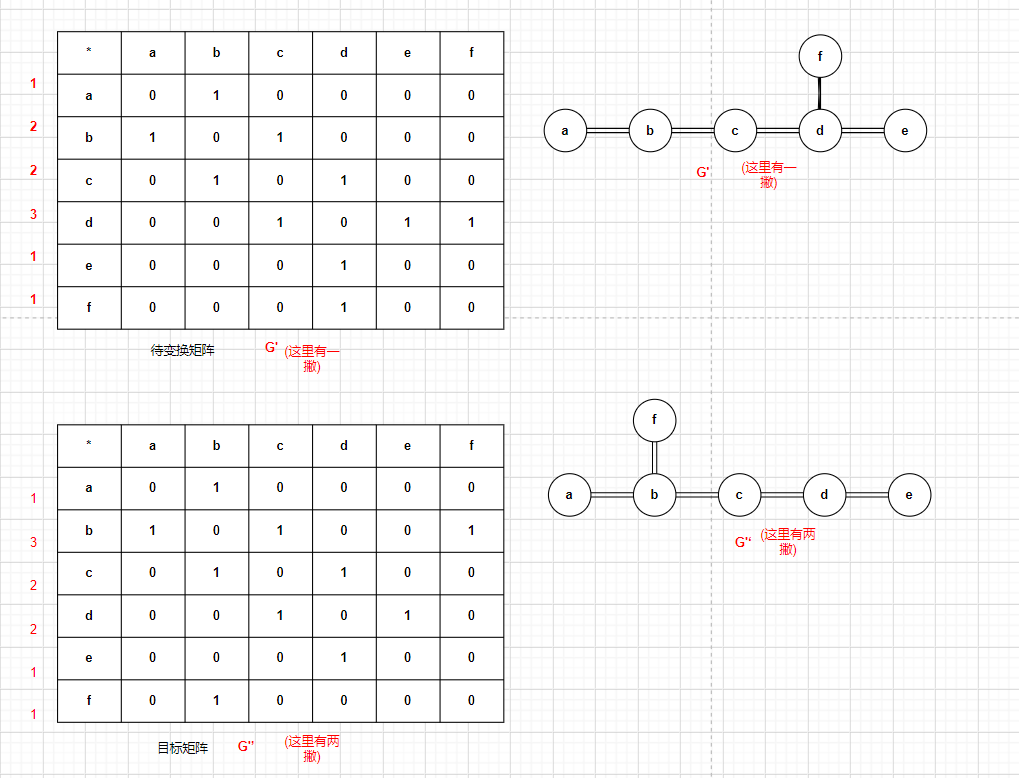矩阵变化流程: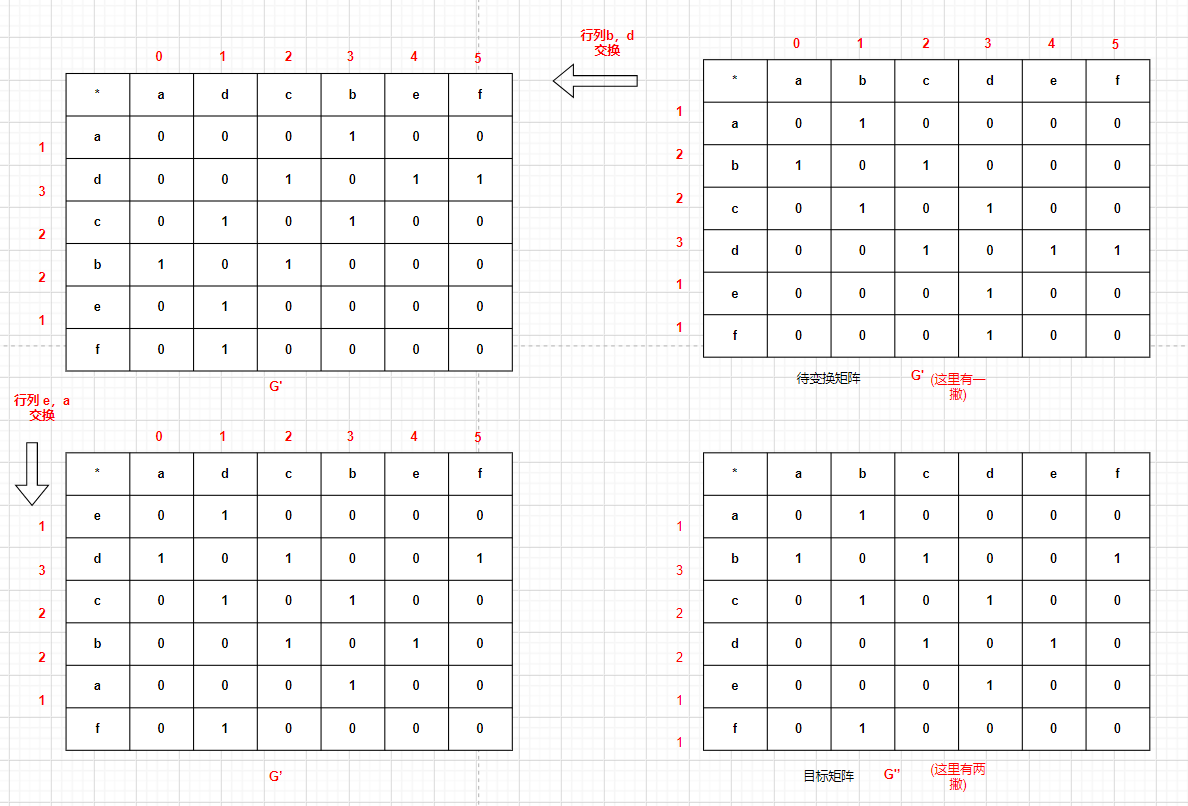测试数据
0 1 0 0 0 0
1 0 1 0 0 0
0 1 0 1 0 0
0 0 1 0 1 1
0 0 0 1 0 0
0 0 0 1 0 0

0 1 0 0 0 0
1 0 1 0 0 1
0 1 0 1 0 0
0 0 1 0 1 0
0 0 0 1 0 0
0 1 0 0 0 0

测试结果：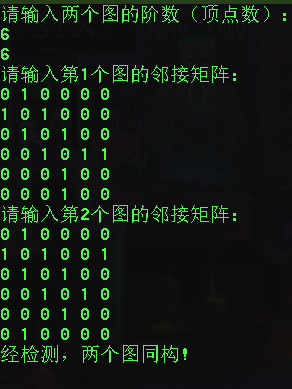测试G和G‘: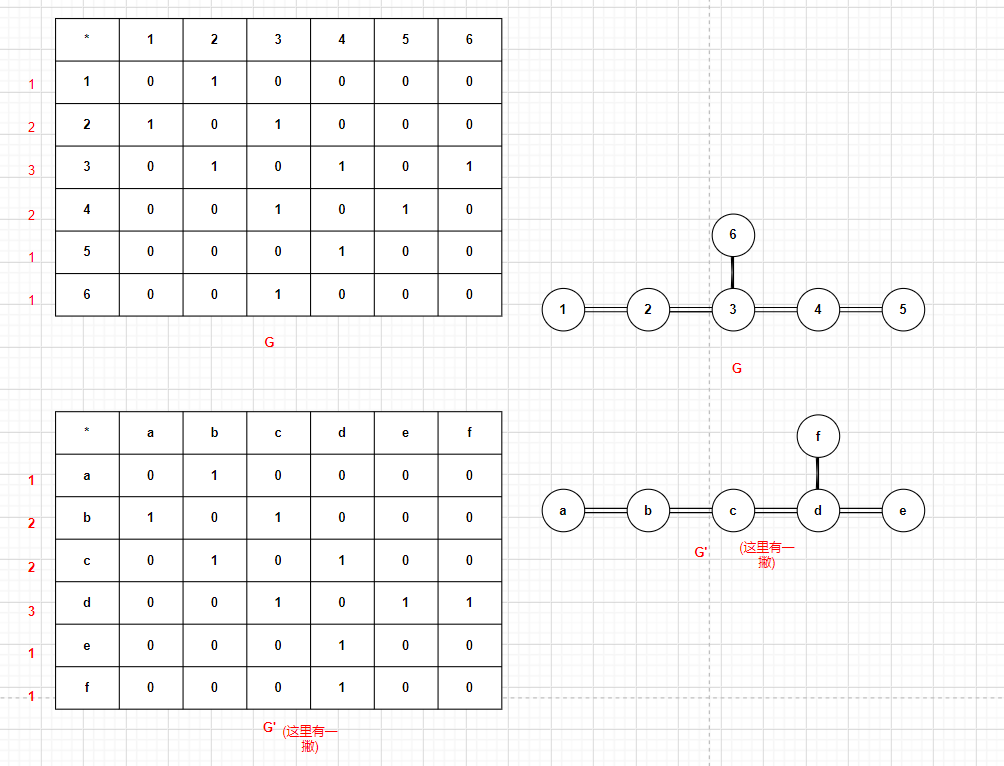测试数据:
0 1 0 0 0 0
1 0 1 0 0 0
0 1 0 1 0 1
0 0 1 0 1 0
0 0 0 1 0 0
0 0 1 0 0 0

0 1 0 0 0 0
1 0 1 0 0 0
0 1 0 1 0 0
0 0 1 0 1 1
0 0 0 1 0 0
0 0 0 1 0 0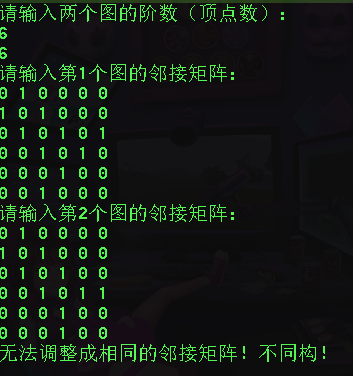算法的时间复杂度和空间复杂度

由代码: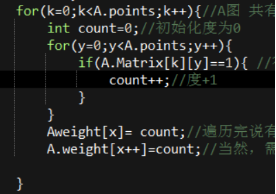O(T1)=N^2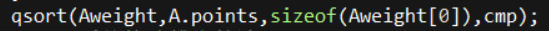O(T2)=N*logN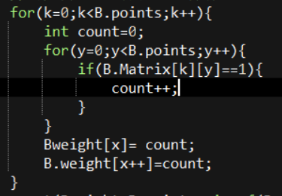O(T3)=N^2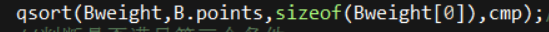O(T4)=N*logN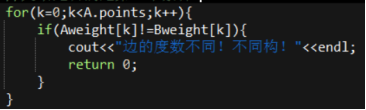O(T5)=N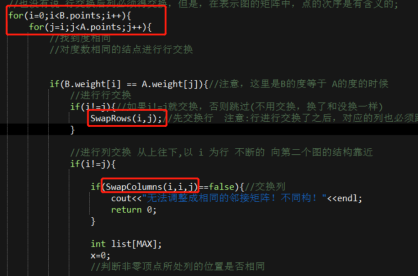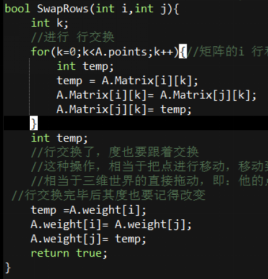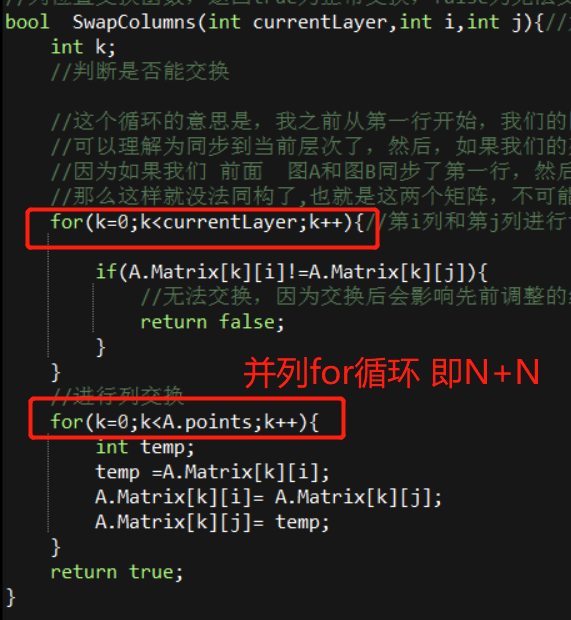O(T6)=N^ 2* N+N^ 2 * (N+N)= N^3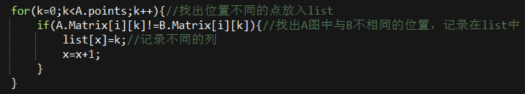O(T7)=N^2 *N=N ^3 //外面有两层for 循环加上这里的就变成N ^3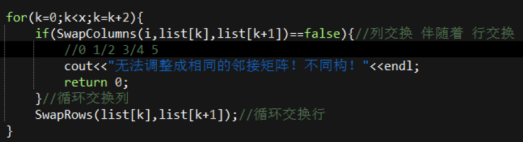假设有k=N ，则这一层的for 循环的执行次数是:N/2
SwapColumns函数若currentLayer为N则SwapColumns的执行次数为:
N+N；
SwapRows函数的执行次数为N;
因此由这一层for循环即 for(k=0;k<x;k=k+2) 的时间复杂度为N/2*(N+N)=N^2
由于外面还有两层for循环,因此这里的时间复杂度为 O(T8)=N^4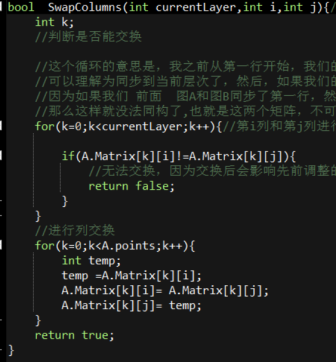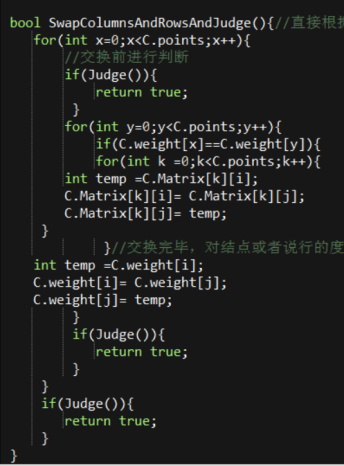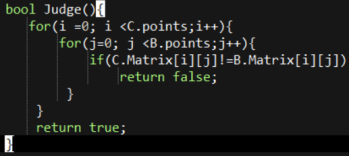O(T9)=N*N^2=N ^3
因此其时间复杂度为:
O(T)=O(T1)+……+O(T9)=N^4
以上两片段 代码还是有问题的，因为没有考虑到全排列这种最坏的情况：
如果矩阵中所有的度都一样，这种情况怎么办呢？直接度相同就交换吗？
改进的代码中 只考虑了度相同就交换，但是，交换后，是不是忽略了什么，比如，其他列的排列情况做变换？即：第一行与另外一度相同的行交换了，那么，除第一行外，其他行都全排列一下才能够遍历所有可能。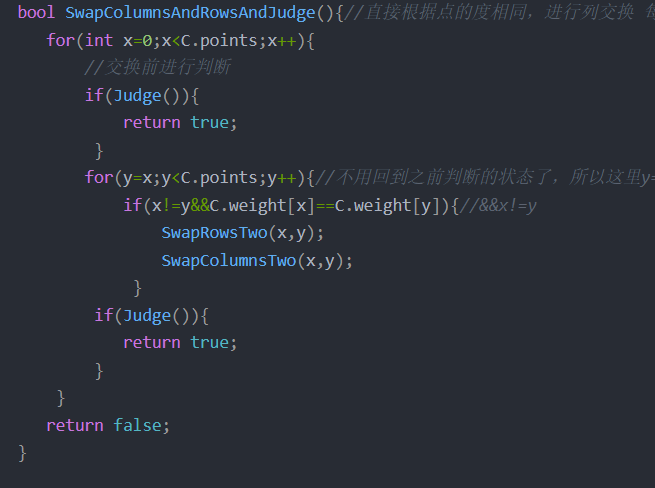仅仅靠两层循环是不能够遍历所有可能的

因此，最后再对代码进行改进，在两个待判定的图的三个必要不充分条件满足后，直接对其中一个矩阵进行全排列，看是否和目标矩阵相同，相同则输出同构，否则输出不同构。

分析出问题，对代码做最后的完善.

全排列判断代码如下：

#include<iostream>
#include<stdlib.h>
#define MAX 100
using namespace std;

int points;             //邻接矩阵的顶点个数（即矩阵阶数）
int edges;              //邻接矩阵的边的条数（即邻接矩阵非零点个数/2）
int Matrix[MAX][MAX];   //矩阵
int weight[MAX];        //行和度数的集合
};

int i,j,k,y,p=0;
//三个必要条件 ① 结点数相同 ②边数相同 ③ 度数相同的结点数相同
// (行进行交换)
//行位置交换函数，返回true为正常交换,这里的行列交换都是针对于图A的
bool SwapRows(int i,int j){//改进代码
int k;
for(k=0;k<A.points;k++){//矩阵的i 行和j行进行交换
int temp;
temp = A.Matrix[i][k];
A.Matrix[i][k]= A.Matrix[j][k];
A.Matrix[j][k]= temp;
}
int temp;
temp =A.weight[i];
A.weight[i]= A.weight[j];
A.weight[j]= temp;
return true;
}

bool  SwapColumns(int i,int j){//改进代码
int k;
for(k=0;k<A.points;k++){
int temp;
temp =A.Matrix[k][i];
A.Matrix[k][i]= A.Matrix[k][j];
A.Matrix[k][j]= temp;
}
return true;
}
int fac(int x)  //阶乘计算
{
register int i,f=1;

for(i=1;i<=x;i++)
f*=i;

return f;
}

bool Judge(){
for(i =0; i <A.points;i++){
for(j=0; j <B.points;j++){
if(A.Matrix[i][j]!=B.Matrix[i][j])
return false;
}
}
return true;
}

bool permutation( int k, int m,int total_number)
{
int i, j;
if (k == m)//k==m的时候就是一种可能
{
++p;
if(p==total_number){
return false;
}
}
else
{
for (j = k; j <= m; j++)
{
SwapRows(j,k);
SwapColumns(j,k);
if(Judge()){
return true;
}
permutation( k + 1, m,total_number);
SwapRows(j,k);//换回来
SwapColumns(j,k);//换回来，在前面变换的基础上继续遍历其他可能。
if(p==total_number){
return false;//for循环里也要添加判断
}
}
}
}
//用于快速排序的比较算法
int cmp( const void *a , const void *b ){
return *(int *)a - *(int *)b;
}

int main(){
cout<<"请输入两个图的阶数（顶点数）："<<endl;
cin>>A.points>>B.points;
//判断第一个必要条件

int total_number=fac(A.points);//初始化阶乘所需要最大次数的值.
if(A.points!=B.points){
cout<<"阶数不同！不同构！"<<endl;
return 0;
}

cout<<"请输入第1个图的邻接矩阵："<<endl;
A.edges = 0;
B.edges = 0;

//用邻接矩阵方式输入A、B矩阵

for(i=0;i<A.points;i++){
for(j=0;j<A.points;j++){
cin>>A.Matrix[i][j];
if(A.Matrix[i][j]==1){
A.edges++;
}
}
}

cout<<"请输入第2个图的邻接矩阵："<<endl;
for(i=0;i<B.points;i++){
for(j=0;j<B.points;j++){
cin>>B.Matrix[i][j];
if(B.Matrix[i][j]==1){
B.edges++;
}
}
}

//判断第二个必要条件

if(A.edges!=B.edges){
cout<<"边的条数不同！不同构！"<<endl;
return 0;
}

//因为是邻接矩阵，所以边的条数（即邻接矩阵非零点个数/2）
//在给边进行赋值的时候，我们在二维 矩阵的值是1的时候都给边+1了，因为是无向图，G[i][j]和G[j][i] 是一样的，因此要/2
A.edges =A.edges/2;
B.edges =B.edges/2;
int Aweight[MAX];//MAX==100
int Bweight[MAX];

//判断第三个必要条件
int x=0;
for(k=0;k<A.points;k++){//A图 共有 point 个点，然后对这些点的度数进行计算
int count=0;//初始化度为0
for(y=0;y<A.points;y++){
if(A.Matrix[k][y]==1){ //有边，度+1,这里不用考虑 要/2 ,因为是针对当前点k而言的,A.Matrix[k][y]==1就说明 k对于y点(变点)而言有边
count++;//度+1
}
}
Aweight[x]= count;//遍历完说有点，统计度后,将其记录在一个一维数组中
x=x+1;
}
qsort(Aweight,A.points,sizeof(Aweight),cmp);
//调用系统快速排序算法
//进行排序的意义是: 因为 第一个点的度是不确定的，因此，我们值能将这个数组进行从小到大（或者从大到小）进行排序，排序完后，数组就是有规律的了
//然后将 B图 记录 点度数的数组也进行从小到大（或者从大到小）进行排序，排序完后，看是否满足 :
//同构图的三个必要条件中的第三个条件:度数相同的节点个数相同

x=0;
//对矩阵B也进行相同的操作
for(k=0;k<B.points;k++){
int count=0;
for(y=0;y<B.points;y++){
if(B.Matrix[k][y]==1){
count++;
}
}
Bweight[x]= count;
B.weight[x++]=count;
}
qsort(Bweight,B.points,sizeof(Bweight),cmp);//调用系统快速排序算法
//判断是否满足第三个条件
for(k=0;k<A.points;k++){
if(Aweight[k]!=Bweight[k]){
cout<<"边的度数不同！不同构！"<<endl;
return 0;
}
}
//矩阵可能一开始就是同构的，然后，因为点的次序不同，而导致矩阵不相同，此时，我们只需要将矩阵进行行列交换，遍历所有的可能，看是否能够达到两个矩阵相同的效果
if(permutation(0,(A.points-1),total_number)){//矩阵全排列
cout<<"经检测，两个图同构!"<<endl;
}else{
cout<<"不同构！"<<endl;
}
return 0;
}

测试样例：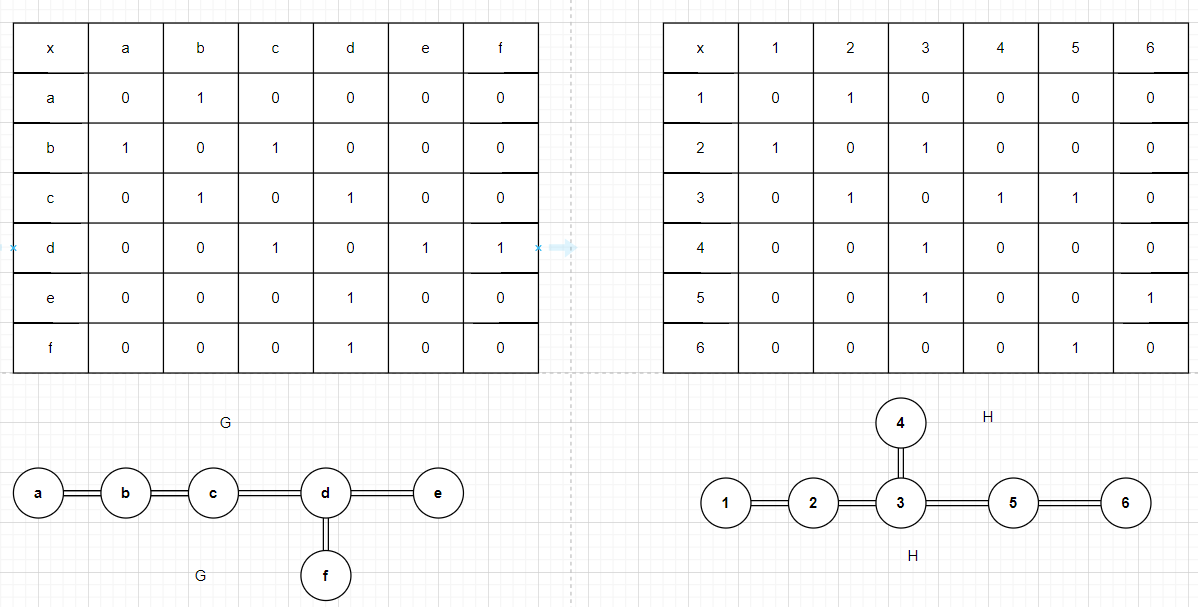测试数据:
0 1 0 0 0 0
1 0 1 0 0 0
0 1 0 1 0 0
0 0 1 0 1 1
0 0 0 1 0 0
0 0 0 1 0 0
………………………
0 1 0 0 0 0
1 0 1 0 0 0
0 1 0 1 1 0
0 0 1 0 0 0
0 0 1 0 0 1
0 0 0 0 1 0
测试结果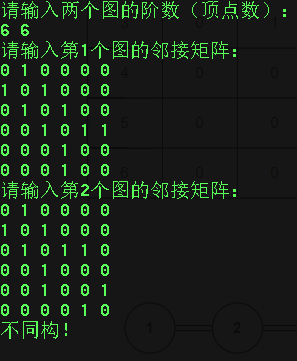再测试一下前面测试过的G和G’测试数据
0 1 0 0 0 0
1 0 1 0 0 0
0 1 0 1 0 0
0 0 1 0 1 1
0 0 0 1 0 0
0 0 0 1 0 0
……………………
0 1 0 0 0 0
1 0 1 0 0 1
0 1 0 1 0 0
0 0 1 0 1 0
0 0 0 1 0 0
0 1 0 0 0 0
测试结果: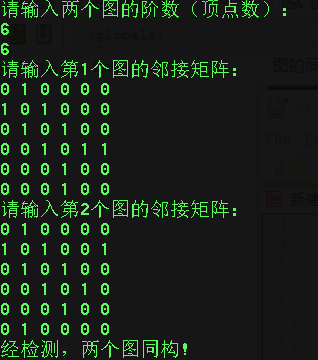因为考虑到矩阵的比较和全排列问题(这才是最坏的情况) 矩阵比较:两个矩阵每个元素都比较一遍 需要N^2，因此，每比较一次都 是N ^2 全排列: 让矩阵行列变化，遍历所有的可能(最坏的情况),也就是N! (N的阶乘)

因此

其时间复杂度为O(T)=N^2 *N!

空间复杂度:
O(T)=N^2

(这下是完全没问题啦!)
😉😀😊

参考博客:

参考博客

展开全文weixin_46607539 2021-11-26 10:45:39
• networkx 判断两图是否同构 得到同构的节点映射 python networkx 同构 映射

qq_34687559 2021-11-11 10:43:45
• networkx 判断异质图同构 前端 networkx 同构

qq_34687559 2021-12-06 11:22:55
• 图的同构 (Graph Isomorphism) mysql数据同构

weixin_39582737 2021-03-04 03:12:07
• PAT树的同构 真·C++实现 c++ 数据结构

Moonlightbear 2021-09-24 10:58:04
• weixin_42208901 2021-01-14 17:29:09
• frank_haha 2021-09-15 14:57:25
• 图论中的概念（3）——同构 抽象代数

scienceknight 2021-01-12 21:19:38
• Java 同构数的问题 java同构数

weixin_34817716 2021-02-12 11:01:22
• 讨论：如何理解同构 同构

m0_37738114 2021-02-23 19:18:55
• weixin_39603327 2021-01-28 21:17:26
• 2021秋季《离散数学》_图的度与同构 算法

rd142857 2021-12-04 20:08:16
• 模拟匹配和同构匹配的区别 人工智能

m0_38013741 2021-04-01 10:52:19
• 树哈希判断同构&无根同构问题转有根同构问题 树结构

BUG_Creater_jie 2021-08-17 00:54:33
• 同构与异构 java sql mysql 数据库 mybatis

terrychinaz 2021-02-08 13:06:17
• 同构字符 算法

qq_15026001 2020-12-22 09:51:17
• weixin_33138295 2021-05-24 09:45:50
• weixin_39689428 2021-01-14 17:24:59
• 字符串循环同构问题与最小表示法 算法

qq_36222817 2021-03-28 15:18:10
• leetcode 96 不同的二叉搜索树: 同构问题分析 卡特兰数 Catalan Number 动态规划 leetcode 算法

u010180372 2021-11-15 09:13:41
• qq_33450671 2021-03-24 10:11:57
• qq_43940950 2021-05-31 11:50:50
• weixin_39610785 2021-02-28 14:26:08
• weixin_33782854 2021-05-20 19:54:37
• 判断同构数C语言 同构数怎么判断

weixin_42372963 2021-02-05 08:11:46
• 群论：同构 与 同态 （群同构 与 群同态） 线性代数 数学

a493823882 2021-12-14 16:20:54
• 统计同构数关键算法 java同构数

weixin_42118701 2021-02-12 11:01:20...